# Guitar Chords Level 2: Enrichment in the Key of G

When learning chords in level 1 we simply learned the 3 most common chords in each of the 7 guitar friendly keys. In level 2 we will learn to enrich the same chords using notes from the major scale (diatonic notes) that are not in the chords we learned.

In level 1, we didn’t discuss how chords are constructed. Questions such as, what does major or minor mean or, why does D7 have a 7 were not discussed. The purpose of level 1 was simply to get you familiar with basic chords. To advance we need to understand basic theory and chord construction.

Do, re, mi, fa, sol, la, ti, do… we have all heard this melody if we were schooled in the western hemisphere. The reason our music teachers sang this melody to us is because these 7 notes are the building blocks of music. These 7 notes are known as the major scale. (How scales are constructed is discussed in my first book, so I won’t discuss that in this lesson). Each note of the major scale is given a number name called intervals: do (1), re (2), mi (3), fa (4), sol (5), la (6), ti (7), do (8). Notice 1 and 8 are the same note. We call the 8 the octave. Chords are constructed by using specific notes from this scale. The first chord we learned is G Major. (The word major is assumed.  Therefore, G major is simply referred to as G). The Major chord is constructed by playing the 1, 3, and 5 intervals simultaneously (in harmony). These 3 intervals create a major triad, the smallest form of a chord. A chord has a minimum of 3 notes.

In the ex.1 we have a diagram of the G major scale. R (red note) designates the root. The root is the 1 interval and it identifies the scale (the G note). The 3 and the 5 are the black dots. They are the remaining notes of the major triad (1,3,5). Learn to play this scale forward and backwards identifying the intervals as you play.

##### * Pay no attention to the p or Δ in the images. That is another lesson.

ex.1 one octave G major scale (intervals)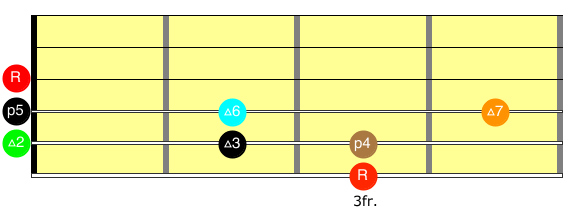In the ex. 2 we have the same scale but the numbers tell us which fingers to use on our left hand. Please use the correct fingers. It is much easier to use good technique from the start than it is breaking bad habits down the road.

ex.2 one octave G major scale (fingers)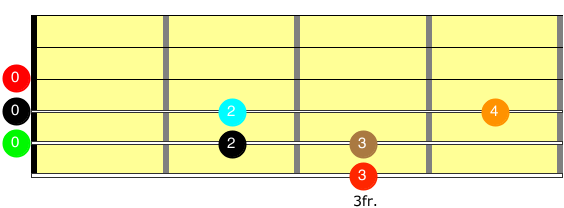In ex. 3 we have the same scale with the notes designated. While learning the notes is essential, I will be emphasizing intervals more in this series of lessons.

ex.3 1 one octave G major scale (notes)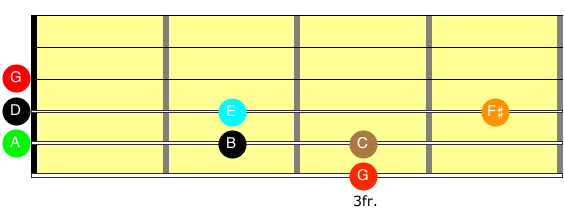ex.4 two octave major scale (intervals)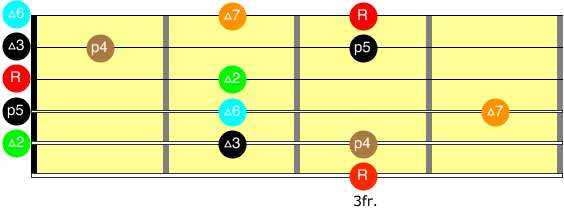I can not over emphasize how important it is to identify intervals of the scale. It is the code, the key, the trick, or the essence of understanding music.

In ex.5 we see the 2 octave major scale again, but with extensions past 8. You can see that the 9 interval is located on the 2 fret, 3 string.

ex. 5 extensions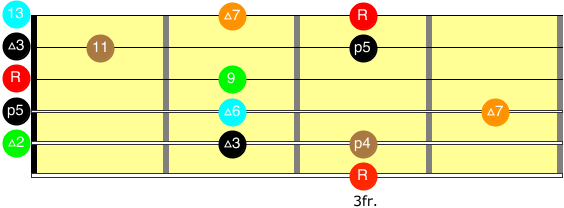Ex.6 illustrates the G chord. Notice we are only playing the roots (1), 3, and 5 intervals (red and black dots only). Again, remember, the major triad has a 1, 3, and 5. We are playing 6 strings but, we are doubling the 3 interval (5 and 2 strings) and we have the root on 3 strings (1, 3, and 6 strings). We only have 3 notes.

##### *The numbers in the far right column are your left hand fingers. The letters are your notes.

When enriching chords we add notes of the scale to the major triad. The intervals of the scale that we have not used are the 2, 4, 6, and 7. The first enriched chord we are going to learn is the Gadd9. Whaaaaat?!!! I thought there were only 7 notes in the scale?! Please, stay with me… yes, there are only 7 notes in the scale. However, a 2 octave major scale has the same notes an octave higher. For example, the 7 notes of the G major scale are G(1), A(2), B(3), C(4), D(5), E(6), F#(7) G(8). Notice that 1 and 8 are the same notes. The notes (letter names) start over. But, the intervals can remain sequential. So, a 2 octave scale can look like this: G(1), A(2), B(3), C(4) D(5), E(6), F#(7), G(1 or 8), A(2 or 9), B(3 or 10), C(4 or 11), D(5 or 12), E(6 or 13), F#(7 or 14). Before we go further, let me point out that you will never use a 10, 12, or 14 interval, so erase those intervals from your memory now. But, you can see how 9 is the same note as 2 but, it is an octave higher. That is my main point! The Gadd9 chord has the intervals 1, 3, 5 (the major triad) and 9. That is why it is called a Gadd9. The reason we use a 9 instead of a 2 is because the root and 2 are very close to each other. This creates dissonance and is not pleasing to our ears. On the other hand, the 9 sounds good.

So, we can take any major chord and add the 9 to get the add9 chord i.e. Gadd9. We can do the same with the 4, 6, and 7 to get our enriched chords. In ex. 7 we will take the G chord and add the other intervals to create enriched chords. Notice the fingerings vary to accommodate the chords.

ex7 Gadd 9, sus, 6, maj7

So in the pervious example we use all 7 notes on the G major scale to enrich the G major chord. Now we will move on to the C major chord. The intervals of the C major chord are derived from the C major scale. In ex. 8 we have the C major scale with the intervals designated.

ex.8 C major scale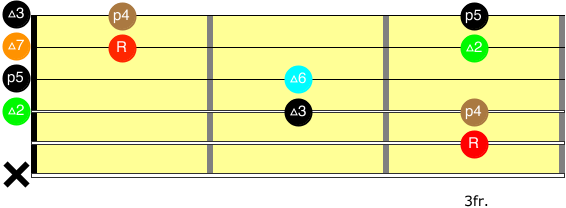In ex. 9 we take the C chord and add the other intervals to create enriched chords. Notice the fingerings vary  to accommodate the chords.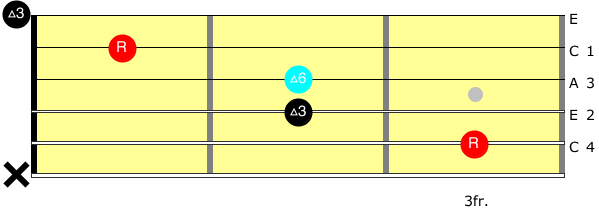Let’s move on to the D7 chord. The intervals are derived from the D major scale shown in ex. 10.

ex.10 D major scale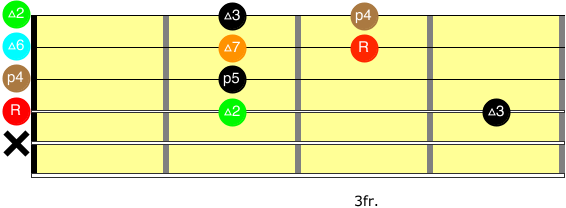Now we will talk about the D7 chord. What I am about to tell you is very important. The D7  is not a triad. It has 4 notes. It has a 1, 3, 5, and ♭7. Although we identify the intervals as they relate to the scale i.e. D7 chord and D major scale, the 7 interval of the D major scale is NOT in the D7 chord. This has been a source of confusion for many. Remember, the major 7 (Maj7) chord has the 1, 3, 5, 7 intervals. There are several symbols used for this chord. They all mean the same thing. GMaj7, GΔ7, and GΔ designate G Major7. The intervals are 1, 3, 5, 7. Again, where the confusion comes in is… D DOMINANT 7, MORE COMMONLY KNOWN AS D7, DOES NOT HAVE A 7! (totally logical right?) D7 has 1, 3, 5 and♭7!!! Briefly, the reason why, is because our harmony is derived from the C scale, which has the C note, from the C scale, not the C# note, from the D scale and a C note is a ♭7 in relation to the D major scale. There is a detailed discussion of this subject in level 3.

In ex. 11 we take the D7 chord and add the other intervals to create enriched chords.

ex.11 D7, 9, 7sus, 13

As you can see, it is impossible to talk about chords in detail without discussing scales. The notes of the chords are derived from the scales. Understanding scales and intervals is essential. Learn to identify each interval by name.

Summary:

We have taken the first 3 chords we learned in level 1, the key of G, which has G, C, and D7. We have used all the notes in the diatonic major scale. 1, 3, 5 is the major triad. We added the 2 (9) to get the add9 chord (1,3,5,9). We added a 4 to get the suspended 4 (1,4,5). We added the 6 to get the 6 chord (1,3,5,6). Lastly, we added the 7 to get major 7 (1,3,5,7). As I mentioned earlier, there are 7 keys in level 1. Likewise, there are 7 keys in level 2 also. After becoming acquainted with the key of G, we can move on to the other 6 keys.

There it is! I hope you enjoy. As always, contact me if you have questions  at guitar lessons by brian.com.

Review for students:

1. Play the G major scale.
2. Play the C major scale
3. Play the D major scale
4. Play Gadd9, Gsus, G6, Gmaj7.
5. Play Cadd9, Csus, C6, Cmaj7.
6. Play D7, D9, D7sus, D13